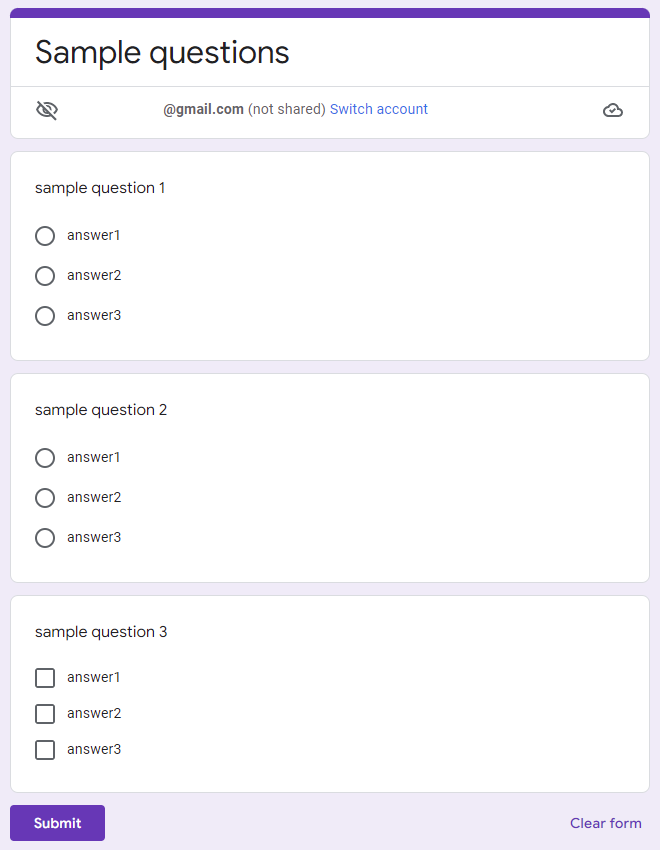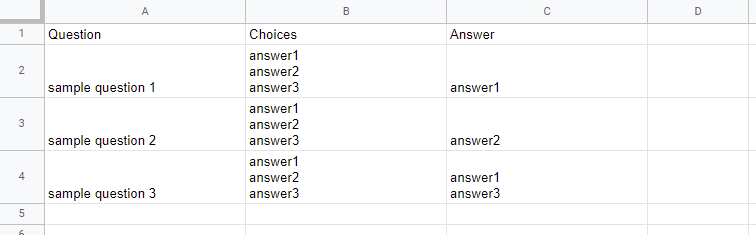GistsThis is a sample script for creating quizzes in Google Form using Google Forms Service with Google Apps Script.

## Usage

### 1. Prepare questions and answers.

In this sample, the questions and answers are prepared using Spreadsheet as follows.### 2. Sample script.

This script is container-bound script of the above Spreadsheet.

function myFunction() {
const formTitle = "sample"; // This is a form title.
const sheetName = "Sheet1"; // This is a sheet name.

const [, ...values] = sheet
.getDataRange()
.getDisplayValues()
.filter((r) => r.join("") != "");
const obj = values.map(([a, b, c]) => {
.split("\n")
.map((e) => e.trim())
.filter(String);
const correct = c
.split("\n")
.map((e) => e.trim())
.filter(String);
return {
question: a,
correct,
point: 1,
};
});
const form = FormApp.create(formTitle)
.setIsQuiz(true)
.setTitle("Sample questions");
obj.forEach(({ question, answers, correct, point, type }) => {
const choice = form[type]();
choice.createChoice(e, correct.includes(e) ? true : false)
);
choice.setTitle(question).setPoints(point).setChoices(choices);
});
}

• When this script is run using the prepared Spreadsheet, you can see the result Google Form at the top image in this post.

## Note

• This sample script doesn’t include the error procession. So please add it for your actual situation.

• I have already published a method for creating this Google Form using Google Forms API at here.